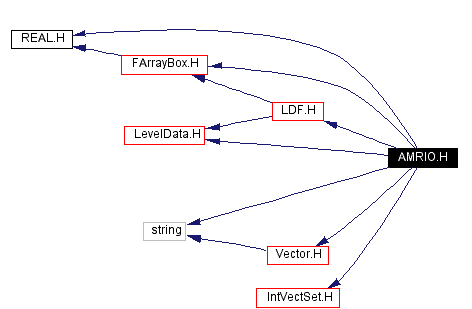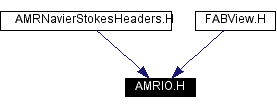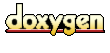Main Page   Namespace List   Class Hierarchy   Alphabetical List   Compound List   File List   Compound Members   File Members

# AMRIO.H File Reference

#include "REAL.H"
#include "LevelData.H"
#include "Vector.H"
#include "FArrayBox.H"
#include "IntVectSet.H"
#include <string>
#include "LDF.H"

Include dependency graph for AMRIO.H:This graph shows which files directly or indirectly include this file:Go to the source code of this file.

class  AMRData

## Functions

void WriteAMRHierarchyHDF5 (string filename, const AMRData &data)
void WriteAMRHierarchyHDF5 (const string &filename, const Vector< DisjointBoxLayout > &a_vectGrids, const Vector< LevelData< FArrayBox > * > &a_vectData, const Vector< string > &a_vectNames, const Box &a_domain, const Real &a_dx, const Real &a_dt, const Real &a_time, const Vector< int > &a_vectRatio, const int &a_numLevels)
void WriteAMRHierarchyHDF5 (HDF5Handle &handle, const Vector< DisjointBoxLayout > &a_vectGrids, const Vector< LevelData< FArrayBox > * > &a_vectData, const Vector< string > &a_vectNames, const Box &a_domain, const Real &a_dx, const Real &a_dt, const Real &a_time, const Vector< int > &a_vectRatio, const int &a_numLevels)
void WriteAMRHierarchyHDF5 (const string &filename, const Vector< DisjointBoxLayout > &a_vectGrids, const Vector< LevelData< FArrayBox > * > &a_vectData, const Box &a_domain, const Vector< int > &a_vectRatio, const int &a_numLevels)
void WriteAMRHierarchyHDF5 (HDF5Handle &handle, const Vector< DisjointBoxLayout > &a_vectGrids, const Vector< LevelData< FArrayBox > * > &a_vectData, const Box &a_domain, const Vector< int > &a_vectRatio, const int &a_numLevels)
int ReadAMRHierarchyHDF5 (string filename, AMRData &data)
int ReadAMRHierarchyHDF5 (const string &filename, Vector< DisjointBoxLayout > &a_vectGrids, Vector< LevelData< FArrayBox > * > &a_vectData, Vector< string > &a_vectNames, Box &a_domain, Real &a_dx, Real &a_dt, Real &a_time, Vector< int > &a_refRatio, int &a_numLevels, const IntVect &a_ghostVector=IntVect::TheZeroVector())
int ReadAMRHierarchyHDF5 (HDF5Handle &handle, Vector< DisjointBoxLayout > &a_vectGrids, Vector< LevelData< FArrayBox > * > &a_vectData, Vector< string > &a_vectNames, Box &a_domain, Real &a_dx, Real &a_dt, Real &a_time, Vector< int > &a_refRatio, int &a_numLevels, const IntVect &a_ghostVector=IntVect::TheZeroVector())
int ReadAMRHierarchyHDF5 (const string &filename, Vector< DisjointBoxLayout > &a_vectGrids, Vector< LevelData< FArrayBox > * > &a_vectData, Box &a_domain, Vector< int > &a_refRatio, int &a_numLevels, const IntVect &a_ghostVector=IntVect::TheZeroVector())
int ReadAMRHierarchyHDF5 (HDF5Handle &handle, Vector< DisjointBoxLayout > &a_vectGrids, Vector< LevelData< FArrayBox > * > &a_vectData, Box &a_domain, Vector< int > &a_refRatio, int &a_numLevels, const IntVect &a_ghostVector=IntVect::TheZeroVector())
int ReadEBAMRASCII (const string &filename, Vector< DisjointBoxLayout > &a_vectGrids, Vector< LevelData< FArrayBox > * > &a_vectData, Vector< string > &a_vectNames, Box &a_domain, Vector< IntVectSet > &a_coveredCells, Vector< IntVectSet > &a_multiValuedCells, Vector< int > &a_refRatio, int &a_numLevels, const IntVect &a_ghostVector=IntVect::TheZeroVector())
void writeFAB (const FArrayBox *a_dataPtr)
void viewFAB (const FArrayBox *a_dataPtr)
void writeFABname (const FArrayBox *a_dataPtr, const char *a_filename)
void writeLDF (const LDF *a_dataPtr)
void writeLevel (const LevelData< FArrayBox > *a_dataPtr)
void viewLevel (const LevelData< FArrayBox > *a_dataPtr)
void writeLDFname (const LDF *a_dataPtr, const string &a_filename)
void writeLevelname (const LevelData< FArrayBox > *a_dataPtr, const char *a_filename)
void WritePartialAMRHierarchyHDF5 (const string &filename, const Vector< DisjointBoxLayout > &a_vectGrids, const Vector< LevelData< FArrayBox > * > &a_vectData, const Vector< string > &a_vectNames, const Box &a_baseDomain, const Real &a_baseDx, const Real &a_dt, const Real &a_time, const Vector< int > &a_vectRatio, const Interval &a_levels)
void writeAFabASCII (std::ostream &os, const FArrayBox &fab)
void readAFabASCII (istream &is, FArrayBox &fab)
void WriteAMRHierarchyASCII (const string &filename, const Vector< DisjointBoxLayout > &a_vectGrids, const Vector< LevelData< FArrayBox > * > &a_vectData, const Vector< string > &a_vectNames, const Box &a_domain, const Real &a_dx, const Real &a_dt, const Real &a_time, const Vector< int > &a_vectRatio, const int &a_numLevels)
int ReadAMRHierarchyASCII (const string &filename, Vector< DisjointBoxLayout > &a_vectGrids, Vector< LevelData< FArrayBox > * > &a_vectData, Vector< string > &a_vectNames, Box &a_domain, Real &a_dx, Real &a_dt, Real &a_time, Vector< int > &a_vectRatio, int &a_numLevels, const IntVect &ghostVector=IntVect::TheZeroVector())

## Function Documentation

 void readAFabASCII ( istream & is, FArrayBox & fab )

 int ReadAMRHierarchyASCII ( const string & filename, Vector< DisjointBoxLayout > & a_vectGrids, Vector< LevelData< FArrayBox > * > & a_vectData, Vector< string > & a_vectNames, Box & a_domain, Real & a_dx, Real & a_dt, Real & a_time, Vector< int > & a_vectRatio, int & a_numLevels, const IntVect & ghostVector = IntVect::TheZeroVector() )
 Reads hierarchy of levels in ASCII format. Returns 0 on success. {\bf Arguments:}\ filename : file to output to.\ a_vectGrids : grids at each level.\ a_vectData : data at each level.\ a_vectNames: names of variables.\ a_domain : domain at coarsest level.\ a_dx : grid spacing at coarsest level.\ a_dt : time step at coarsest level.\ a_time : time.\ a_vectRatio : refinement ratio at all levels (ith entry is refinement ratio between levels i and i + 1).\ a_numLevels : number of levels to output.\ a_ghostVector : number of ghost cells on output .\ {\bf Returns:}\ status code with values:\ 0: success\ -1: failure\

 int ReadAMRHierarchyHDF5 ( HDF5Handle & handle, Vector< DisjointBoxLayout > & a_vectGrids, Vector< LevelData< FArrayBox > * > & a_vectData, Box & a_domain, Vector< int > & a_refRatio, int & a_numLevels, const IntVect & a_ghostVector = IntVect::TheZeroVector() )
 Reads hierarchy of levels in HDF5 format. Only available if the preprocessor macro HDF5 is defined at compilation. Returns 0 on success. {\bf Arguments:}\ handle : handle to input from.\ a_vectGrids : grids at each level.\ a_vectData : data at each level.\ a_domain : domain at coarsest level.\ a_vectRatio : refinement ratio at all levels (ith entry is refinement ratio between levels i and i + 1).\ a_numLevels : number of levels to read.\ {\bf Returns:} \ status code with values:\ 0: success \ -1: bogus number of levels \ -2: bogus number of components \ -3: error in readlevel \ -4: file open failed \ This is not blocking.

 int ReadAMRHierarchyHDF5 ( const string & filename, Vector< DisjointBoxLayout > & a_vectGrids, Vector< LevelData< FArrayBox > * > & a_vectData, Box & a_domain, Vector< int > & a_refRatio, int & a_numLevels, const IntVect & a_ghostVector = IntVect::TheZeroVector() )
 Reads hierarchy of levels in HDF5 format. Only available if the preprocessor macro HDF5 is defined at compilation. Returns 0 on success. {\bf Arguments:}\ filename : file to input from.\ a_vectGrids : grids at each level.\ a_vectData : data at each level.\ a_domain : domain at coarsest level.\ a_vectRatio : refinement ratio at all levels (ith entry is refinement ratio between levels i and i + 1).\ a_numLevels : number of levels to read.\ {\bf Returns:} \ status code with values:\ 0: success \ -1: bogus number of levels \ -2: bogus number of components \ -3: error in readlevel \ -4: file open failed \ This is blocking.

 int ReadAMRHierarchyHDF5 ( HDF5Handle & handle, Vector< DisjointBoxLayout > & a_vectGrids, Vector< LevelData< FArrayBox > * > & a_vectData, Vector< string > & a_vectNames, Box & a_domain, Real & a_dx, Real & a_dt, Real & a_time, Vector< int > & a_refRatio, int & a_numLevels, const IntVect & a_ghostVector = IntVect::TheZeroVector() )
 Reads hierarchy of levels in HDF5 format. Only available if the preprocessor macro HDF5 is defined at compilation. Returns 0 on success. {\bf Arguments:}\ handle : handle to input from.\ a_vectGrids : grids at each level.\ a_vectData : data at each level.\ a_vectNames: names of variables.\ a_domain : domain at coarsest level.\ a_dx : grid spacing at coarsest level.\ a_dt : time step at coarsest level.\ a_time : time.\ a_vectRatio : refinement ratio at all levels (ith entry is refinement ratio between levels i and i + 1).\ a_numLevels : number of levels to read.\ {\bf Returns:} \ status code with values:\ 0: success \ -1: bogus number of levels \ -2: bogus number of components \ -3: error in readlevel \ -4: file open failed \ This is not blocking

 int ReadAMRHierarchyHDF5 ( const string & filename, Vector< DisjointBoxLayout > & a_vectGrids, Vector< LevelData< FArrayBox > * > & a_vectData, Vector< string > & a_vectNames, Box & a_domain, Real & a_dx, Real & a_dt, Real & a_time, Vector< int > & a_refRatio, int & a_numLevels, const IntVect & a_ghostVector = IntVect::TheZeroVector() )
 Reads hierarchy of levels in HDF5 format. Only available if the preprocessor macro HDF5 is defined at compilation. Returns 0 on success. {\bf Arguments:}\ filename : file to input from.\ a_vectGrids : grids at each level.\ a_vectData : data at each level.\ a_vectNames: names of variables.\ a_domain : domain at coarsest level.\ a_dx : grid spacing at coarsest level.\ a_dt : time step at coarsest level.\ a_time : time.\ a_vectRatio : refinement ratio at all levels (ith entry is refinement ratio between levels i and i + 1).\ a_numLevels : number of levels to read.\ {\bf Returns:} \ status code with values:\ 0: success \ -1: bogus number of levels \ -2: bogus number of components \ -3: error in readlevel \ -4: file open failed \ This is blocking

 int ReadAMRHierarchyHDF5 ( string filename, AMRData & data )
 Reads hierarchy of levels in HDF5 format. Only available if the preprocessor macro HDF5 is defined at compilation. Returns 0 on success. {\bf Arguments:}\ filename : file to input from.\ a_vectGrids : grids at each level.\ a_vectData : data at each level.\ a_vectNames: names of variables.\ a_domain : domain at coarsest level.\ a_dx : grid spacing at coarsest level.\ a_dt : time step at coarsest level.\ a_time : time.\ a_vectRatio : refinement ratio at all levels (ith entry is refinement ratio between levels i and i + 1).\ a_numLevels : number of levels to read.\ {\bf Returns:} \ status code with values:\ 0: success \ -1: bogus number of levels \ -2: bogus number of components \ -3: error in readlevel \ -4: file open failed \ This is blocking

 int ReadEBAMRASCII ( const string & filename, Vector< DisjointBoxLayout > & a_vectGrids, Vector< LevelData< FArrayBox > * > & a_vectData, Vector< string > & a_vectNames, Box & a_domain, Vector< IntVectSet > & a_coveredCells, Vector< IntVectSet > & a_multiValuedCells, Vector< int > & a_refRatio, int & a_numLevels, const IntVect & a_ghostVector = IntVect::TheZeroVector() )
 Reads hierarchy of EB levels in ASCII format. Returns 0 on success. Data is read in as FArrayBoxes because the data file does not include sufficient information to reconstruct the EBIS. {\bf Arguments:}\ filename : file to input from.\ a_vectGrids : grids at each level.\ a_vectData : data at each level.\ a_vectNames: names of variables.\ a_domain : domain at coarsest level.\ a_vectRatio : refinement ratio at all levels (ith entry is refinement ratio between levels i and i + 1).\ a_multiValuedCells : multi-valued cells at all levels \ (ith entry is refinement ratio between levels i and i + 1).\ a_coveredCells : covered cells at all levels \ (ith entry is refinement ratio between levels i and i + 1).\ a_numLevels : number of levels to read.\ returns 0 if successful.

 void viewFAB ( const FArrayBox * a_dataPtr )
 View *a_dataPtr by writing it to an HDF5 plotfile (to a temporary file) and then running ChomboVis with a python script which brings up a data browser by default. The file has the same format as WriteAMRHierarchyHDF5, but for a single FArrayBox. This is useful for debugging.

 void viewLevel ( const LevelData< FArrayBox > * a_dataPtr )
 View *a_dataPtr by writing it to an HDF5 plotfile (to a temporary file) and then running ChomboVis with a python script which brings up a data browser by default. The file has the same format as WriteAMRHierarchyHDF5, but for a single LevelData. This is useful for debugging.

 void writeAFabASCII ( std::ostream & os, const FArrayBox & fab )

 void WriteAMRHierarchyASCII ( const string & filename, const Vector< DisjointBoxLayout > & a_vectGrids, const Vector< LevelData< FArrayBox > * > & a_vectData, const Vector< string > & a_vectNames, const Box & a_domain, const Real & a_dx, const Real & a_dt, const Real & a_time, const Vector< int > & a_vectRatio, const int & a_numLevels )
 Writes hierarchy of levels in ASCII format. {\bf Arguments:}\ filename : file to output to.\ a_vectGrids : grids at each level.\ a_vectData : data at each level.\ a_vectNames: names of variables.\ a_domain : domain at coarsest level.\ a_dx : grid spacing at coarsest level.\ a_dt : time step at coarsest level.\ a_time : time.\ a_vectRatio : refinement ratio at all levels (ith entry is refinement ratio between levels i and i + 1).\ a_numLevels : number of levels to output.\

 void WriteAMRHierarchyHDF5 ( HDF5Handle & handle, const Vector< DisjointBoxLayout > & a_vectGrids, const Vector< LevelData< FArrayBox > * > & a_vectData, const Box & a_domain, const Vector< int > & a_vectRatio, const int & a_numLevels )
 Writes hierarchy of levels in HDF5 format. Only available if the preprocessor macro HDF5 is defined at compilation. {\bf Arguments:}\ handle : handle to output to.\ a_vectGrids : grids at each level.\ a_vectData : data at each level.\ a_domain : domain at coarsest level.\ a_vectRatio : refinement ratio at all levels (ith entry is refinement ratio between levels i and i + 1).\ a_numLevels : number of levels to output.\ This is not blocking.

 void WriteAMRHierarchyHDF5 ( const string & filename, const Vector< DisjointBoxLayout > & a_vectGrids, const Vector< LevelData< FArrayBox > * > & a_vectData, const Box & a_domain, const Vector< int > & a_vectRatio, const int & a_numLevels )
 Writes hierarchy of levels in HDF5 format. Only available if the preprocessor macro HDF5 is defined at compilation. {\bf Arguments:}\ filename : file to output to.\ a_vectGrids : grids at each level.\ a_vectData : data at each level.\ a_domain : domain at coarsest level.\ a_vectRatio : refinement ratio at all levels (ith entry is refinement ratio between levels i and i + 1).\ a_numLevels : number of levels to output.\ This is blocking.

 void WriteAMRHierarchyHDF5 ( HDF5Handle & handle, const Vector< DisjointBoxLayout > & a_vectGrids, const Vector< LevelData< FArrayBox > * > & a_vectData, const Vector< string > & a_vectNames, const Box & a_domain, const Real & a_dx, const Real & a_dt, const Real & a_time, const Vector< int > & a_vectRatio, const int & a_numLevels )
 Writes hierarchy of levels in HDF5 format. Only available if the preprocessor macro HDF5 is defined at compilation. {\bf Arguments:}\ handle : handle to output to.\ a_vectGrids : grids at each level.\ a_vectData : data at each level.\ a_vectNames: names of variables.\ a_domain : domain at coarsest level.\ a_dx : grid spacing at coarsest level.\ a_dt : time step at coarsest level.\ a_time : time.\ a_vectRatio : refinement ratio at all levels (ith entry is refinement ratio between levels i and i + 1).\ a_numLevels : number of levels to output.\ This is not blocking.

 void WriteAMRHierarchyHDF5 ( const string & filename, const Vector< DisjointBoxLayout > & a_vectGrids, const Vector< LevelData< FArrayBox > * > & a_vectData, const Vector< string > & a_vectNames, const Box & a_domain, const Real & a_dx, const Real & a_dt, const Real & a_time, const Vector< int > & a_vectRatio, const int & a_numLevels )
 Writes hierarchy of levels in HDF5 format. Only available if the preprocessor macro HDF5 is defined at compilation. {\bf Arguments:}\ filename : file to output to.\ a_vectGrids : grids at each level.\ a_vectData : data at each level.\ a_vectNames: names of variables.\ a_domain : domain at coarsest level.\ a_dx : grid spacing at coarsest level.\ a_dt : time step at coarsest level.\ a_time : time.\ a_vectRatio : refinement ratio at all levels (ith entry is refinement ratio between levels i and i + 1).\ a_numLevels : number of levels to output.\ This is blocking.

 void WriteAMRHierarchyHDF5 ( string filename, const AMRData & data )
 Writes hierarchy of levels in HDF5 format. Only available if the preprocessor macro HDF5 is defined at compilation. {\bf Arguments:}\ filename : file to output to.\ a_vectGrids : grids at each level.\ a_vectData : data at each level.\ a_vectNames: names of variables.\ a_domain : domain at coarsest level.\ a_dx : grid spacing at coarsest level.\ a_dt : time step at coarsest level.\ a_time : time.\ a_vectRatio : refinement ratio at all levels (ith entry is refinement ratio between levels i and i + 1).\ a_numLevels : number of levels to output.\ This is blocking.

 void writeFAB ( const FArrayBox * a_dataPtr )
 Writes a plotfile using the same format as WriteAMRHierarchyHDF5, but for a FArrayBox. This is useful for debugging. *a_dataPtr is written to a file named fab.hdf5

 void writeFABname ( const FArrayBox * a_dataPtr, const char * a_filename )
 Writes a plotfile using the same format as WriteAMRHierarchyHDF5, but for a FArrayBox. This is useful for debugging. *a_dataPtr is written to the file given by a_filename.

 void writeLDF ( const LDF * a_dataPtr )
 Write a plotfile using the same format as WriteAMRHierarchyHDF5, but for a single LevelData. Useful for debugging. *a_dataPtr is written to a file named LDF.hdf5.

 void writeLDFname ( const LDF * a_dataPtr, const string & a_filename )
 Write a plotfile using the same format as WriteAMRHierarchyHDF5, but for a single LevelData. Useful for debugging. *a_dataPtr is written to the file given by a_filename.

 void writeLevel ( const LevelData< FArrayBox > * a_dataPtr )
 Write a plotfile using the same format as WriteAMRHierarchyHDF5, but for a single LevelData. Useful for debugging. *a_dataPtr is written to a file named LDF.hdf5.

 void writeLevelname ( const LevelData< FArrayBox > * a_dataPtr, const char * a_filename )
 Write a plotfile using the same format as WriteAMRHierarchyHDF5, but for a single LevelData. Useful for debugging. *a_dataPtr is written to the file given by a_filename.

 void WritePartialAMRHierarchyHDF5 ( const string & filename, const Vector< DisjointBoxLayout > & a_vectGrids, const Vector< LevelData< FArrayBox > * > & a_vectData, const Vector< string > & a_vectNames, const Box & a_baseDomain, const Real & a_baseDx, const Real & a_dt, const Real & a_time, const Vector< int > & a_vectRatio, const Interval & a_levels )
 Write a plotfile with levels that don't necessarily begin with 0 (will translate things so that lBase comes out as level 0, etc) Levels in Vector arguments should correspond with actual levels. (and so should be defined for a_levels.begin() through a_levels.end(). {\bf Arguments:}\ filename : file to output to.\ a_vectGrids : grids at each level.\ a_vectData : data at each level.\ a_vectNames: names of variables.\ a_domain : domain at base level (given by a_levels.begin()).\ a_dx : grid spacing at base level.\ a_dt : time step at base level.\ a_time : time.\ a_vectRatio : refinement ratio at all levels (ith entry is refinement ratio between levels i and i + 1).\ a_numLevels : number of levels to output.\ This is blocking.

Generated on Thu Aug 29 11:05:47 2002 for Chombo&INS by1.2.16